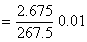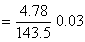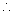# All India Engineering / Architecture Entrance Examination (AIEEE)

AIEEE 2010 Chemistry

Note: Questions with (*) mark are from syllabus of class XI.

Q.1. A solution containing 2.675 g of CoCl3.6NH3 (molar mass = 267.5 g mol-1) is passed through a cation exchanger. The chloride ions obtained in solution were treated with excess of AgNO3 to give 4.78 g of AgCl (molar mass = 143.5 g mol-1). The formula of the complex is (Atomic mass of Ag = 108 u)

1. [Co(NH3)6]Cl3
2. [CoCl2(NH3)4]Cl
3. [CoCl3(NH3)3]
4. [CoCl(NH3)5]Cl2

Sol :

Number of moles of the complexNumber of moles of AgCl formedNumber of moles of AgCl formed = 3 × Number of moles of the complexThe formula of the complex is [Co(NH3)6]Cl3

Q. 2. If 10-4 dm3of water is introduced into 1.0 dm3 flask at 300 K, how many moles of water are in the vapour phase when equilibrium is established? (Given: Vapour pressure of H2O at 300 K is 3170 Pa ; R = 8.314 JK-1 mol-1)

1. 5.56 × 10-3mol
2. 1.53 × 10-2 mol
3. 4.46 × 10-2mol
4. 1.27 × 10-3 mol

Sol :

The volume occupied by water molecules in vapour phase is (1 – 10-4) dm3, that is approximately 1 dm3.